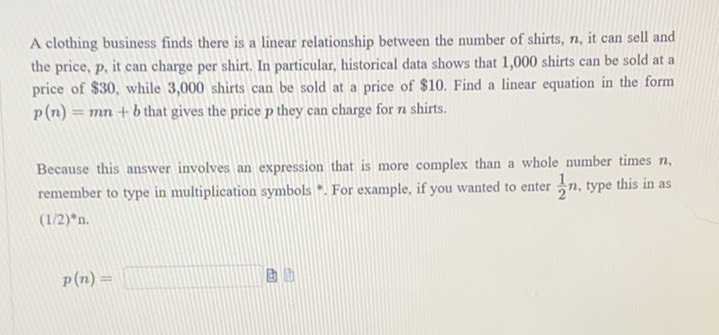### ¿Todavía tienes preguntas de matemáticas?

Pregunte a nuestros tutores expertos
Algebra
PreguntaA clothing business finds there is a linear relationship between the number of shirts, $$n$$ , it can sell and the price, $$p$$ , it can charge per shirt. In particular, historical data shows that $$1,000$$ shirts can be sold at a price of $$\ 30$$ , while $$3,000$$ shirts can be sold at a price of $$\ 10$$ . Find a linear equation in the form

$$p ( n ) = m n + b$$ that gives the price $$p$$ they can charge for $$n$$ shirts. Because this ansiver involves an expression that is more complex than a whole number times $$n$$ , remember to type in multiplication symbols . For example, if you wanted to enter $$\frac { 1 } { 2 } n$$ , type this in as (1/2) $$^ { * } n$$ .